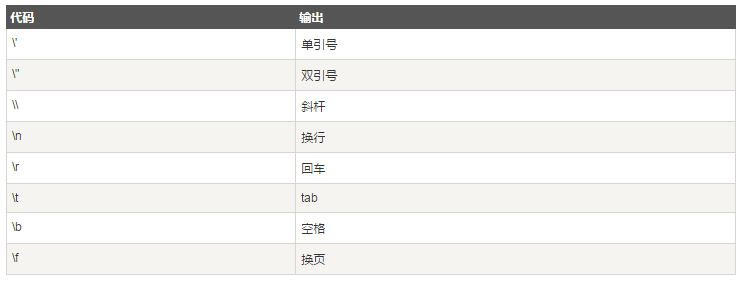# 深入解析JavaScript中的数字对象与字符串对象

JavaScript Number 对象
JavaScript 只有一种数字类型。

JavaScript 数字
JavaScript 数字可以使用也可以不使用小数点来书写：

```var pi=3.14;  // 使用小数点
var x=34;    // 不使用小数点```

```var y=123e5;  // 12300000
var z=123e-5;  // 0.00123```

JavaScript 不是类型语言。与许多其他编程语言不同，JavaScript 不定义不同类型的数字，比如整数、短、长、浮点等等。

`52 bits (0 - 51) 11 bits (50 - 62) 1 bit (63)`

`var x = 0.2+0.1; // result will be 0.30000000000000004`

```var y = 0377;
var z = 0xFF;```

lamp 绝不要在数字前面写零，除非您需要进行八进制转换。

```var myNumber=128;
myNumber.toString(16);  // returns 80
myNumber.toString(8);  // returns 200
myNumber.toString(2);  // returns 10000000```

```myNumber=2;
while (myNumber!=Infinity)
{
myNumber=myNumber*myNumber; // Calculate until Infinity
}
```

```var x = 2/0;
var y = -2/0;```

NaN - 非数字值
NaN 属性是代表非数字值的特殊值。该属性用于指示某个值不是数字。可以把 Number 对象设置为该值，来指示其不是数字值。

```var x = 1000 / "Apple";
isNaN(x); // returns true
var y = 100 / "1000";
isNaN(y); // returns false```

```var x = 1000 / 0;
isNaN(x); // returns false```

JavaScript 数字对象初始化数据， var y = new Number(123);

```var x = 123;
var y = new Number(123);
typeof(x) // returns Number
typeof(y) // returns Object```

```var x = 123;
var y = new Number(123);
(x === y) // is false because x is a number and y is an object.```

JavaScript 字符串（String） 对象
String 对象用于处理已有的字符块。
JavaScript 字符串

```var carname="Volvo XC60";
var carname='Volvo XC60';```

`var character=carname;`

```var answer="It's alright";
var answer="He is called 'Johnny'";
var answer='He is called "Johnny"';```

```var answer='It's alright';
var answer="He is called "Johnny"";```

```var txt="Hello World!";
document.write(txt.length);

var txt="ABCDEFGHIJKLMNOPQRSTUVWXYZ";
document.write(txt.length);
```

```var str="Hello world, welcome to the universe.";
var n=str.indexOf("welcome");
```

lastIndexOf() 方法在字符串末尾开始查找字符串出现的位置。

match()函数用来查找字符串中特定的字符，并且如果找到的话，则返回这个字符。

```var str="Hello world!";
document.write(str.match("world") + "<br>");
document.write(str.match("World") + "<br>");
document.write(str.match("world!"));

```

replace() 方法在字符串中用某些字符替换另一些字符。

```str="Please visit Microsoft!"
var n=str.replace("Microsoft","w3cschool");
```

```var txt="Hello World!";    // String
var txt1=txt.toUpperCase();  // txt1 is txt converted to upper
var txt2=txt.toLowerCase();  // txt2 is txt converted to lower```

```txt="a,b,c,d,e"  // String
txt.split(",");  // Split on commas
txt.split(" ");  // Split on spaces
txt.split("|");  // Split on pipe ```

Javascript 中可以使用反斜线（）插入特殊符号，如：撇号,引号等其他特殊符号。

```var txt="We are the so-called "Vikings" from the north.";
document.write(txt);```

```var txt="We are the so-called "Vikings" from the north.";
document.write(txt);```

JavaScript将输出正确的文本字符串：We are the so-called "Vikings" from the north.JavaScript中Boolean对象的属性解析
constructor属性实例返回通过myvar对象的原型创建的函数：varmyvar=newBoolean(1);myvar.constructor;结果输出：functionBoolean(){[nativecode]}定义和用法constructor属性返回

JavaScript中的所有事物都是对象：字符串、数值、数组、函数...此外，JavaScript允许自定义对象。所有事物都是对象JavaScript提供多个内建对象，比如String

JavaScript中Date对象的常用方法示例
getFullYear()使用getFullYear()获取年份。源代码:/script!DOCTYPEhtmlhtmlbodypid="demo"Clickthebuttontodisplaythefullyearoftodaysdate./pbuttononclick="myFunction()"Tryit/buttonscriptfunctionmyF

Copyright © 2010 Gimoo.Net. All Rights Rreserved  京ICP备05050695号